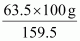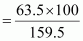# How much copper can be obtained from 100 g of copper sulphate (CuSO4) ?9 years ago

1 mole of CuSO4 contains 1 mole of copper.

Molar mass of CuSO4 = (63.5) + (32.00) + 4(16.00)

= 63.5 + 32.00 + 64.00

= 159.5 g

159.5 g of CuSO4 contains 63.5 g of copper.

⇒ 100 g of CuSO4 will containof copper.

Amount of copper that can be obtained from 100 g CuSO4= 39.81 g